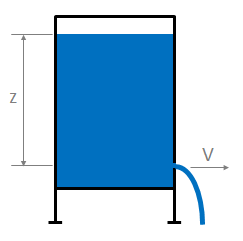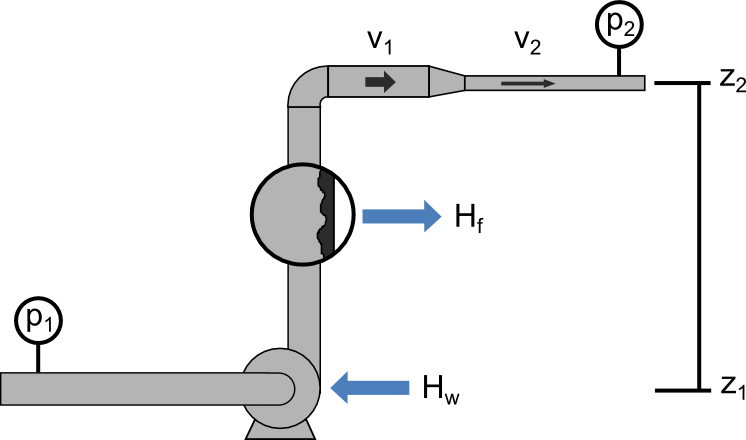f
Neutrium

# Bernoulli’s Equation

Torricelli's LawThis article presents Torricelli’s law, a simplified method of estimating the velocity of fluid passing through an open orifice under static pressure.

Bernoulli's EquationBernoulli’s Principle is an important observation in fluid dynamics which states that for an inviscid flow, an increase in the velocity of the fluid results in a simultaneous decrease in pressure or a decrease in the fluid’s potential energy. This principle is often represented mathematically in the many forms of Bernoulli’s equation. This article presents some useful forms of Bernoulli’s Equations and their simplifying assumptions.